# Minimum number of jumps

Minimum number of jumps: Here, we are going to learn how to find the minimum number of jumps to reach the end of the array (starting from the first element)?
Submitted by Radib Kar, on February 17, 2020

Description:

This problem is a standard interview problem which has been featured in interview rounds of Adobe, Amazon, Oyo rooms etc.

Problem statement:

Given an array of integers where each element represents the max number of steps that can be made forward from that element. The task is to find the minimum number of jumps to reach the end of the array (starting from the first element). If an element is 0, then there is no way to move from there.

```    Input:
The first line is T, total number of test cases.
In the following 2*T lines,
Each test case has two lines.
First line is a number n denoting the size of the array.
Next line contains the sequence of integers a1, a2, ..., an

Output:
For each test case, in a new line,
print the minimum number of jumps.
If it's not possible to reach the end the print -1
```

Example:

```    Input:
1
11
1 3 5 3 1 2 6 0 6 2 4

Output:
4
```

Explanation:

```    Let's first use greedy method.
According to greedy,

At first jump,
it will move from index 0 to 1
(arr=1, so only 1 step jump is allowed)

At second jump,
It will move from index 1 to 4
(arr=3, so only 3 step jump is allowed)

At third jump,
it will move from index 4 to 6
(arr=2, so only 2 step jump is allowed)

Now arr=0, so no more jump possible. It would result -1.

But, it's not the result. Thus, greedy fails.
```

Since, the array value is maximum jump possible we have to check for all options up to maximum jumps. Obviously, a recursive function which would generate many overlapping sub problems. Let's check how we can solve the above example using recursion.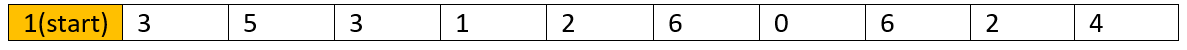After first jump (in this case only one move possible)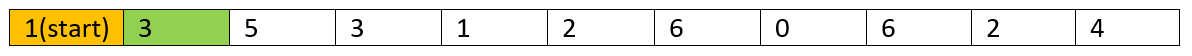After second jump (I only took the best child (on solution path) of the recursion tree, you can try all)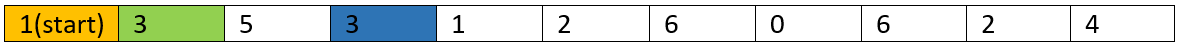After third jump (I only took the best child (on solution path) of the recursion tree, you can try all)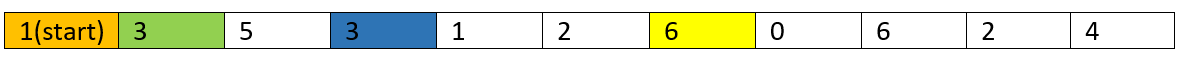After fourth jump (That's end)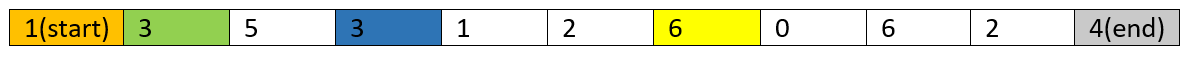Let's check the DP approach to solve the above problem.

Solution approach:

```    1)  If the starting index has value 0 then we can't reach the end
if the array size is more than 1, so return -1.
2)  If array size is 1, we are at the end already, so return 1
which is minimum jump.
3)  Initialize DP[n]  with all elements having value INT_MAX
4)  for i=0 to n-1
for j=i+1 to maximum of(n-1,j+arr[i])
//i.e,last index or upto theindex maximum jump can reach
dp[j]=minimum(dp[j],dp[i]+1)
where dp[i]+1=one jump added with jumps required to reach index i
end for
end for

// not updated in the loop refers that reach end is not possible
5)  if dp[n-1]==INT_MAX
return -1
else
return dp[n-1]
```

C++ Implementation:

```#include <bits/stdc++.h>
using namespace std;

int min(int x,int y){
return (x<y)?x:y;
}

int minimumjumps(vector<int> arr,int n){
if(n==1)
return 1;
if(arr==0)
return -1;

int dp[n];

for(int i=0;i<n;i++)
dp[i]=INT_MAX;
dp=0;
for(int i=0;i<n;i++){
for(int j=i+1;j<=((i+arr[i])>(n-1)?(n-1):(i+arr[i]));j++){
dp[j]=min(dp[j],dp[i]+1);
}
}
return dp[n-1];
}

int main()
{
int t,n,item;

cout<<"output -1 if reaching end not possible\n";
cout<<"Enter number of testcases\n";
scanf("%d",&t);

for(int i=0;i<t;i++){
cout<<"Enter array size\n";
scanf("%d",&n);

vector<int> a;

cout<<"Enter elements:\n";
for(int j=0;j<n;j++){
scanf("%d",&item);
a.push_back(item);
}

int result=minimumjumps(a,n);

cout<<"Result is: ";
if(result==INT_MAX)
cout<<-1<<endl;
else
cout<<result<<endl;
}

return 0;
}
```

Output

```output -1 if reaching end not possible
Enter number of testcases
1
Enter array size
11
Enter elements:
1 3 5 3 1 2 6 0 6 2 4
Result is: 4
```